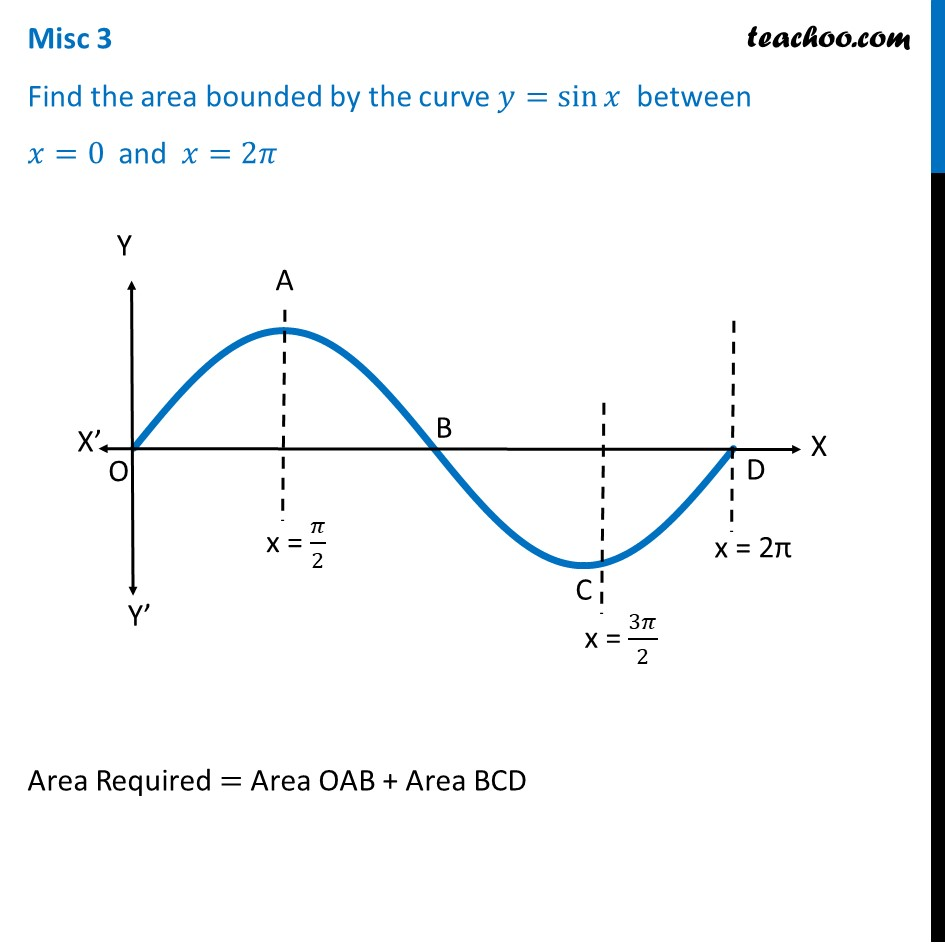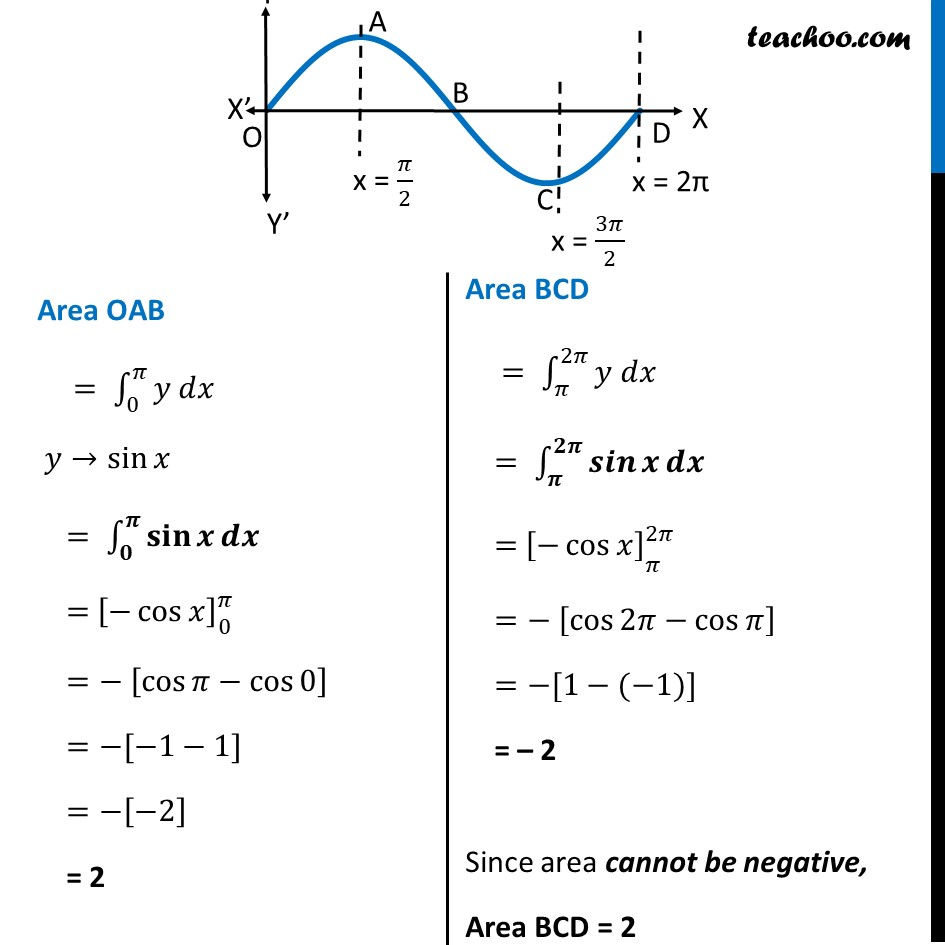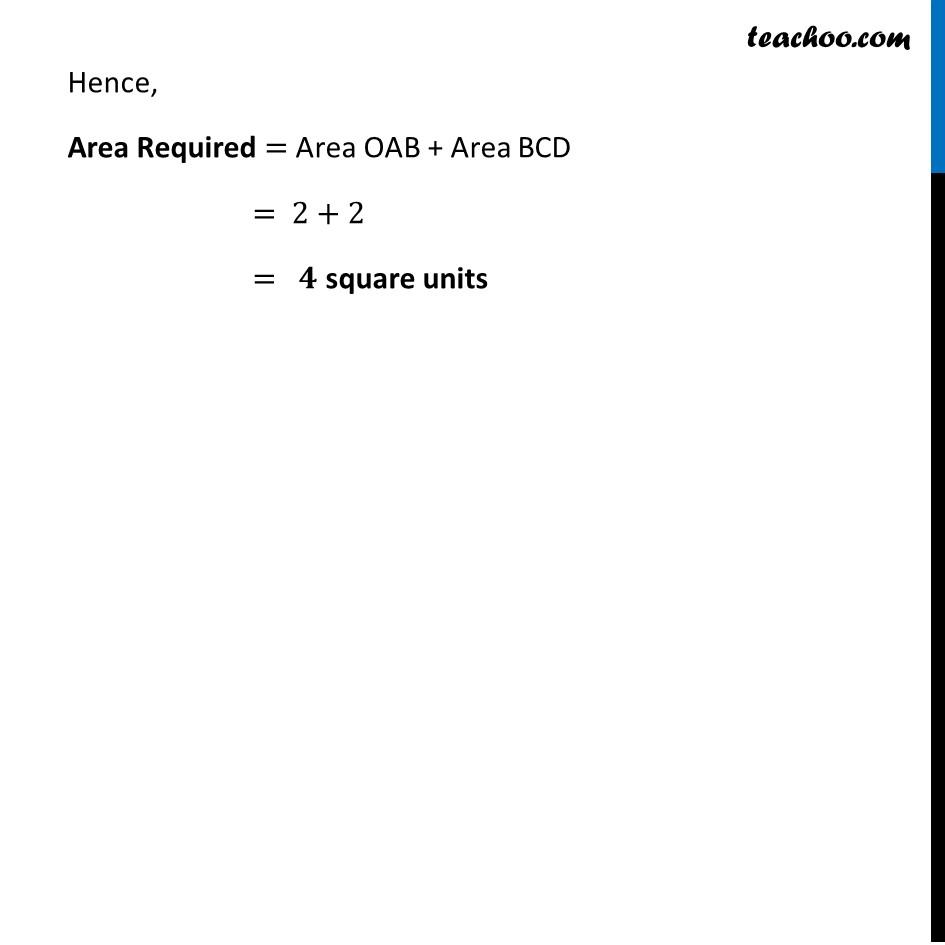Miscellaneous

Chapter 8 Class 12 Application of Integrals
Serial order wiseLearn in your speed, with individual attention - Teachoo Maths 1-on-1 Class

### Transcript

Misc 3 Find the area bounded by the curve 𝑦=sin⁡𝑥 between 𝑥=0 and 𝑥=2𝜋 Area Required = Area OAB + Area BCD Area OAB = ∫_0^𝜋▒〖𝑦 𝑑𝑥〗 𝑦→sin⁡𝑥 = ∫_𝟎^𝝅▒〖𝐬𝐢𝐧⁡𝒙 𝒅𝒙〗 = [−cos⁡𝑥 ]_█( @0)^𝜋 =− [cos⁡𝜋−cos⁡0 ] =−[−1−1] =−[−2] = 2 Area BCD = ∫_𝜋^2𝜋▒〖𝑦 𝑑𝑥〗 = ∫_𝝅^𝟐𝝅▒〖𝒔𝒊𝒏⁡𝒙 𝒅𝒙〗 = [−cos⁡𝑥 ]_█( @ @𝜋)^2𝜋 =− [cos⁡2𝜋−cos⁡𝜋 ] =−[1−(−1)] = – 2 Since area cannot be negative, Area BCD = 2 Hence, Area Required = Area OAB + Area BCD = 2+2 = 𝟒 square units